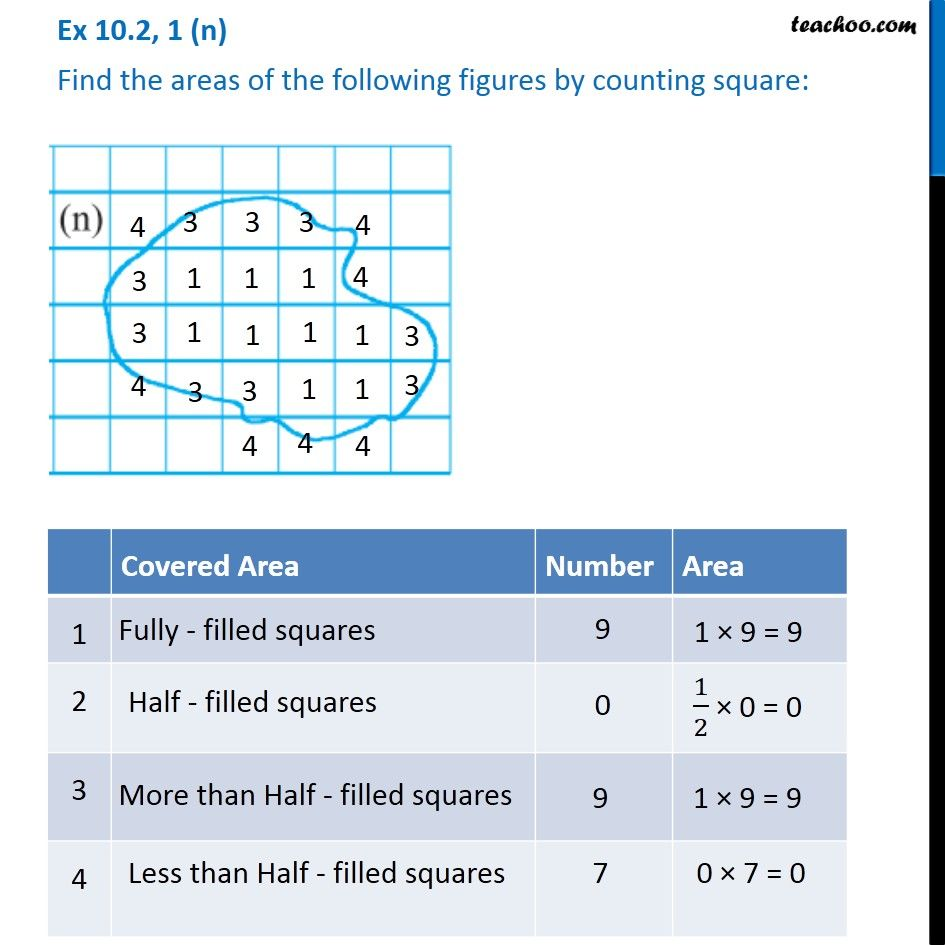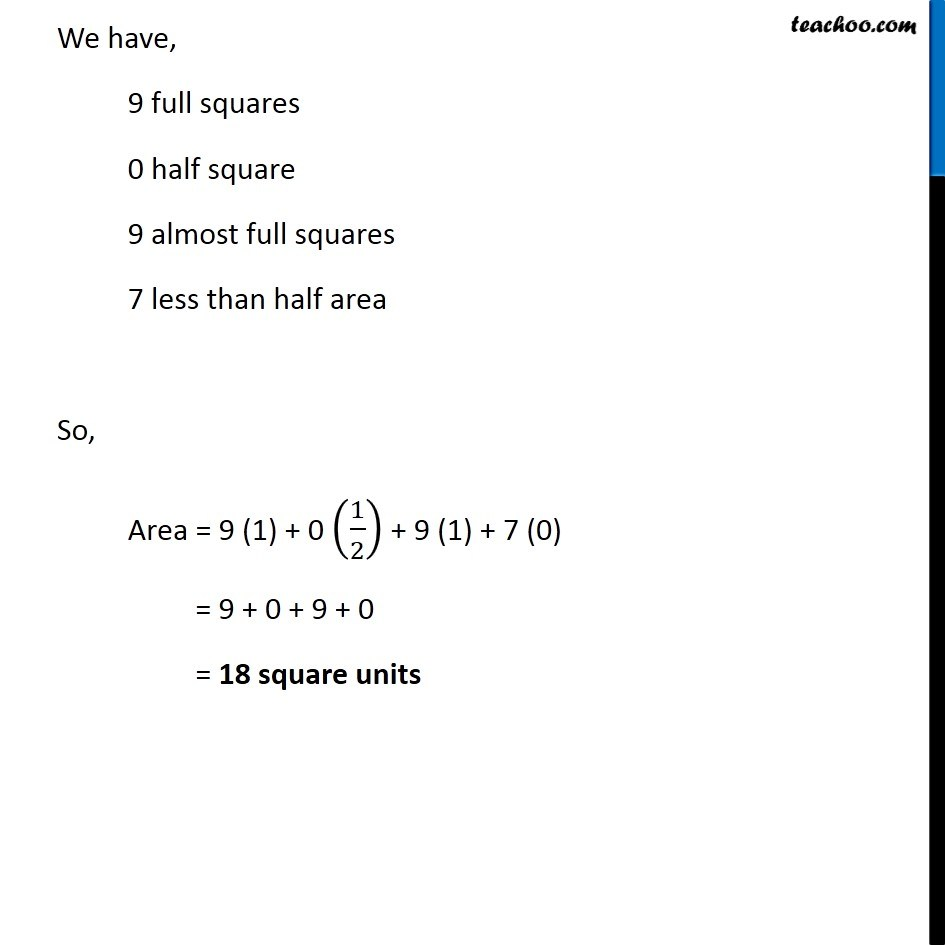1. Chapter 10 Class 6 Mensuration
2. Concept wise
3. Finding area by counting squares

Transcript

Ex 10.2, 1 (n) Find the areas of the following figures by counting square: Covered Area Number Area 1 Fully filled squares 9 1 × 9 = 9 2 Half filled squares 0 1/2 × 0 = 0 3 More than Half filled squares 9 1 × 9 = 9 4 Less than half filled squares 7 0 × 7 = 0 We have, 9 full squares 0 half square 9 almost full squares 7 less than half area So, Area = 9 (1) + 0 (1/2) + 9 (1) + 7 (0) = 9 + 0 + 9 + 0 = 18 square units